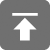# 工学1号馆

home

## C语言动态存储分配

Wu Yudong    June 27, 2015     C   485

C语言支持动态存储分配，即在程序执行期间分配内存单元的能力，利用动态存储分配，可以根据需要设计扩大（或缩小）的数据结构，虽然可以适用于所有类型的数据，但是动态存储分配更常用于字符串、数组和结构体

### 内存分配函数

3种内存分配函数都是声明在<stdlib.h>中：

• malloc函数–分配内存块，但是不对内存块进行初始化
• calloc函数–分配内存块，并且对内存块进行消除
• realloc函数–调整先前分配的内存块

malloc函数不需要对分配的内存快进行清除，所以它比calloc函数更高效

### 空指针

p=malloc(10000);
if(p==NULL) {
/*分配失败*/
}

if(p==NULL)
if(!p)
if(p!=NULL)
if(p)

typedef struct {
long key;
/*...*/
}Record;
float *myFunc(size_t n)
{
double *dptr=malloc(sizeof(double));
if(dptr==NULL)
{
/*...*/
return NULL:
}
else
{
*dptr=0.07;
}
/*...*/
Record *rptr;
if(rptr=malloc(2*sizeof(Record))==NULL)
{
/*...*/
return NULL;
}
float *fptr=malloc(n*sizeof(float));
if(fptr==NULL)
{
/*...*/
return NULL:
}
/*...*/
return fptr;
}

### 动态分配数组

int *a;
a=malloc(n * sizeof(int));

calloc函数

calloc函数在<stdlib.h>中具有如下的原型：

void *calloc(size_t nmemb, size_t size);

a=calloc(n, sizeof(int))

struct point{int x,y;}*p;
p=calloc(1,sizeof(struct point));

realloc函数

realloc函数可以调整数组的大小使它更适合需要，realloc函数原型在<stdlib.h>中：

void *realloc(void *ptr, size_t size)

ptr指向的内存块一定是先前通过malloc函数、calloc函数或realloc函数的调用获得的，size表示内存块的新尺寸

C语言标准列出几条关于realloc函数的规则：

• 当扩展内存块时，realloc函数不会对添加进内存块的自己进行初始化
• 如果realloc函数不能按照要求扩大内存块，那么它会返回空指针，并且在原有的内存块中的数据不会发生改变
• 如果realloc函数调用时以空指针作为第一个实际参数，那么它的行为就像malloc函数一样
• 如果realloc函数调用时以0作为第二个实际参数，那么它会释放掉内存块

### 释放存储

p=malloc(...)
q=malloc(...)
p=q;

free函数在<stdlib.h>中有如下原型：

void free(void *ptr)

p=malloc(...)
q=malloc(...)
free(p);
p=q;